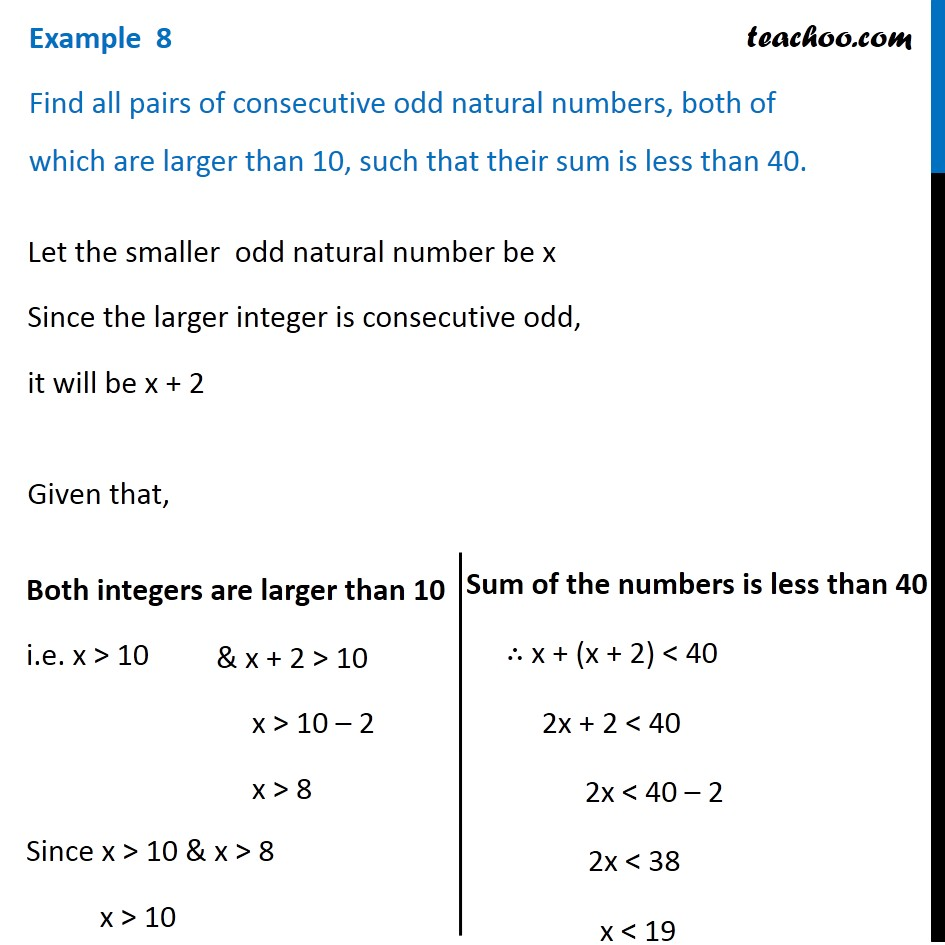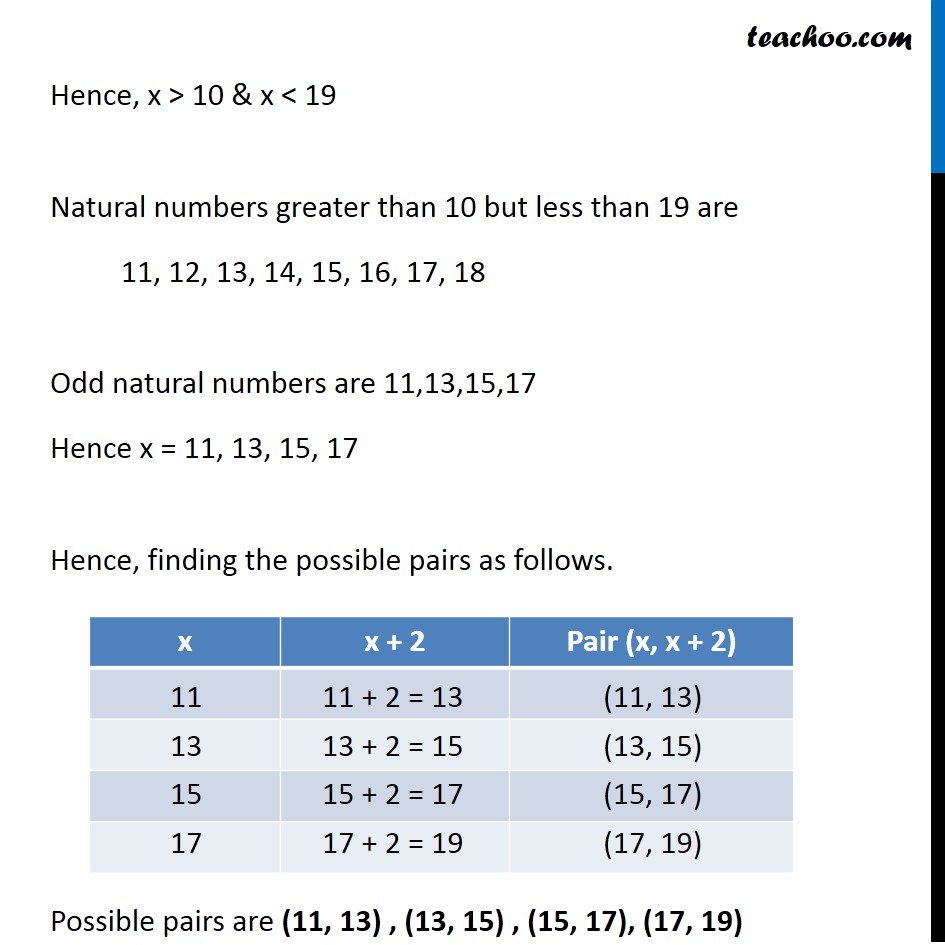Examples

Chapter 5 Class 11 Linear Inequalities
Serial order wiseLearn in your speed, with individual attention - Teachoo Maths 1-on-1 Class

### Transcript

Example 8 Find all pairs of consecutive odd natural numbers, both of which are larger than 10, such that their sum is less than 40. Let the smaller odd natural number be x Since the larger integer is consecutive odd, it will be x + 2 Given that, Both integers are larger than 10 i.e. x > 10 Since x > 10 & x > 8 x > 10 & x + 2 > 10 x > 10 – 2 x > 8 Sum of the numbers is less than 40 ∴ x + (x + 2) < 40 2x + 2 < 40 2x < 40 – 2 2x < 38 x < 19 Hence, x > 10 & x < 19 Natural numbers greater than 10 but less than 19 are 11, 12, 13, 14, 15, 16, 17, 18 Odd natural numbers are 11,13,15,17 Hence x = 11, 13, 15, 17 Hence, finding the possible pairs as follows. Possible pairs are (11, 13) , (13, 15) , (15, 17), (17, 19)